# Graphics¶

WulffPack offers several means to visualize the created particles. This page collects some examples.

## Show a particle with matplotlib¶

The simplest way to show a particle with WulffPack is with the view function. The function uses matplotlib and the user can specify colors of facets, transparency and thickness of the lines between the facets via optional keywords. The keyword save_as specifies the filename to save the figure as. If none is specified, the particle is shown with the matplotlib GUI.

surface_energies = {(1, 1, 1): 1.0,
(1, 0, 0): 1.1,
(1, 1, 0): 1.12}
particle = Decahedron(surface_energies=surface_energies,
twin_energy=0.02)

# Define colors (optional)
colors = {(1, 1, 1): '#7da275',
(1, 0, 0): '#0053b5',
(1, 1, 0): '#f6faf5'}
# Show with GUI:
particle.view()
# Save as file:
particle.view(colors=colors, linewidth=0.3, alpha=0.9,
save_as='single_particle.png')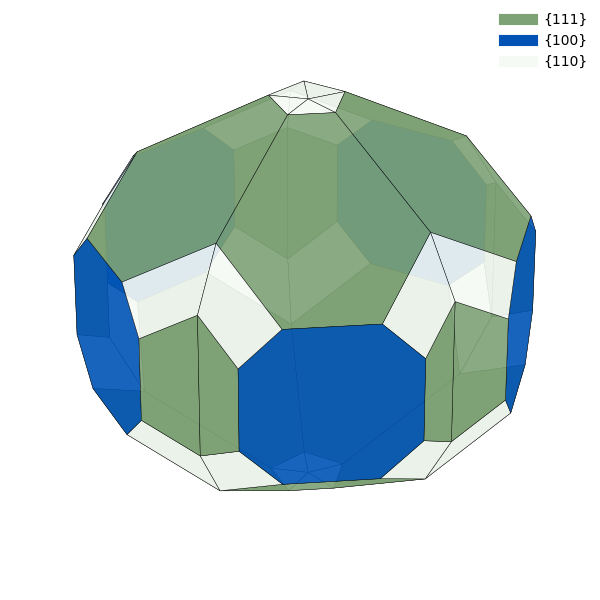If the particle contains vicinal surfaces, it can be advantageous to highlight the vicinality between surfaces with a continuous color scheme, such that surfaces that are similar have similar colors. WulffPack has a function get_continuous_color_scheme, which creates such color schemes automatically. Note that these color schemes are sensible primarily for crystals with cubic symmetry.

surface_energies_vicinal = {(1, 1, 1): 1.0,
(1, 1, 0): 1.1,
(1, 0, 0): 1.1,
(3, 3, 2): 1.05,
(2, 1, 0): 1.15}
particle_vicinal = SingleCrystal(surface_energies_vicinal)
continuous_colors = particle_vicinal.get_continuous_color_scheme()
particle_vicinal.view(colors=continuous_colors)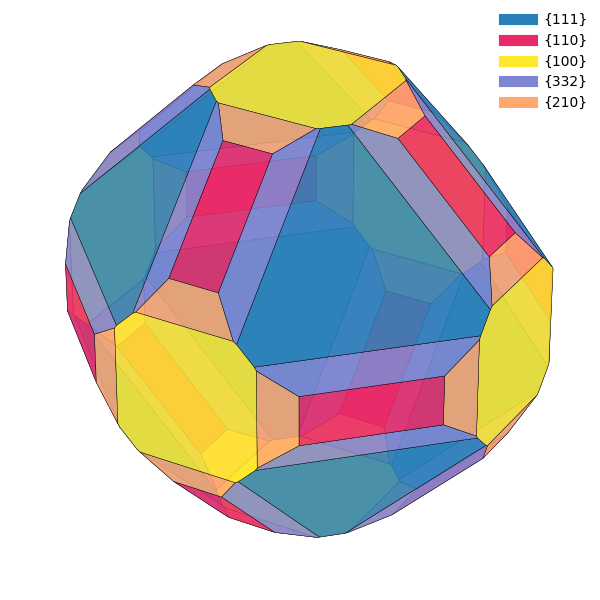## Make subplots¶

For more control over the matplotlib figure, one can also get axis objets directly. In the following snippet, a truncated octahedron, decahedron and icosahedron are created and put as subplots in the same figure.

Note that it can be quite tricky to get 1:1 aspect ratio when plotting 3D with matplotlib. In this example, the figure size is manually set to three times as wide as tall, and the distance from edge of the window to plot as well as between plots is set to zero using subplots_adjust(). This ensures that the plots are not squeezed in any direction.

import matplotlib.pyplot as plt
from mpl_toolkits.mplot3d import Axes3D

fig = plt.figure(figsize=(3 * 4.0, 4.0))
particle = SingleCrystal(surface_energies)
particle.make_plot(ax, colors=colors)

particle = Decahedron(surface_energies,
twin_energy=0.05)
particle.make_plot(ax, colors=colors)

particle = Icosahedron(surface_energies,
twin_energy=0.05)
particle.make_plot(ax, colors=colors)

right=1, wspace=0, hspace=0)
plt.savefig('particles.png')
plt.show()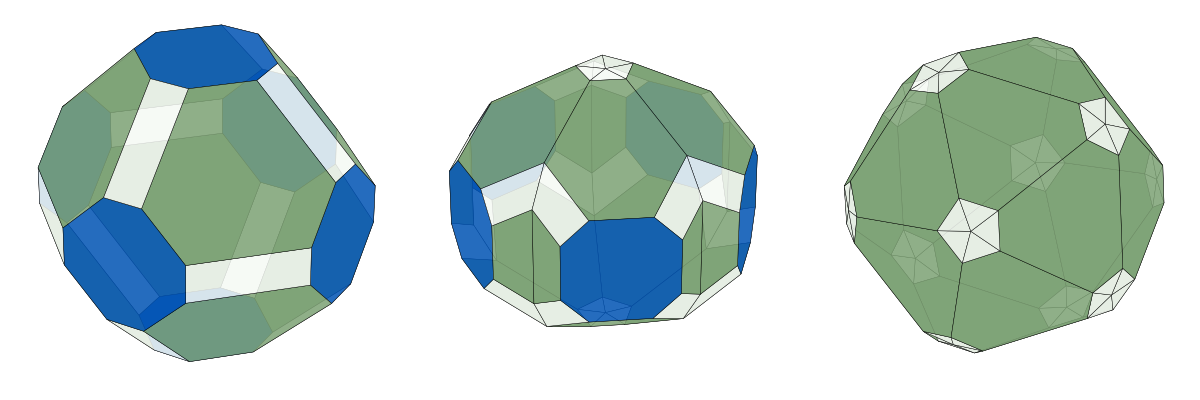## Visualize atoms objects¶

The possibility to extract an ASE Atoms object opens an arsenal of visualization opportunities. The arguably simplest one is ASE’s GUI.

from ase.visualize import view
view(particle.atoms)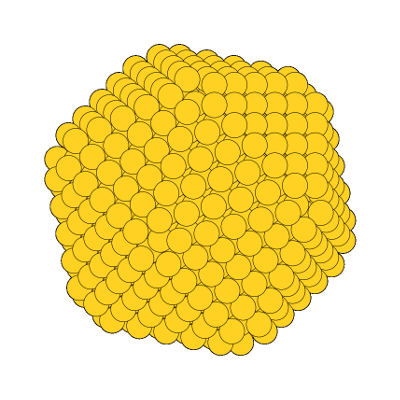A truncated icosahedron visualized with ASE’s GUI.

Since an ASE Atoms object can be written to file, virtually any software that visualizes atomistic structures can be utilized.

from ase.io import write
write('atoms.xyz', particle.atoms)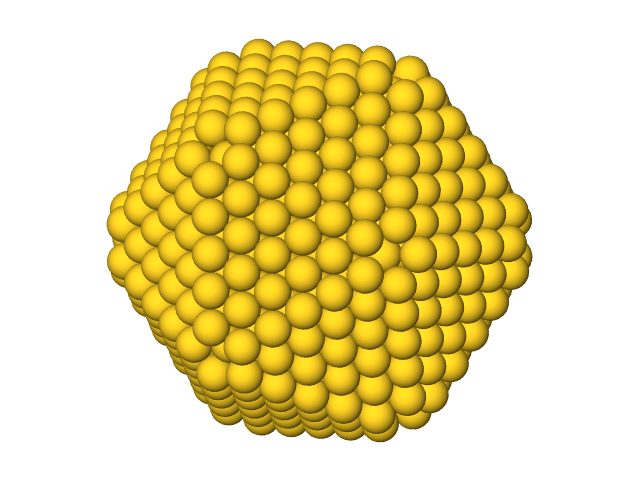A truncated icosahedron visualized with OVITO.

## Use wavefront .obj files¶

The write can write particles to Wavefront .obj files. Such files are useful in many 3D software, such as Blender.

particle.write('particle.obj')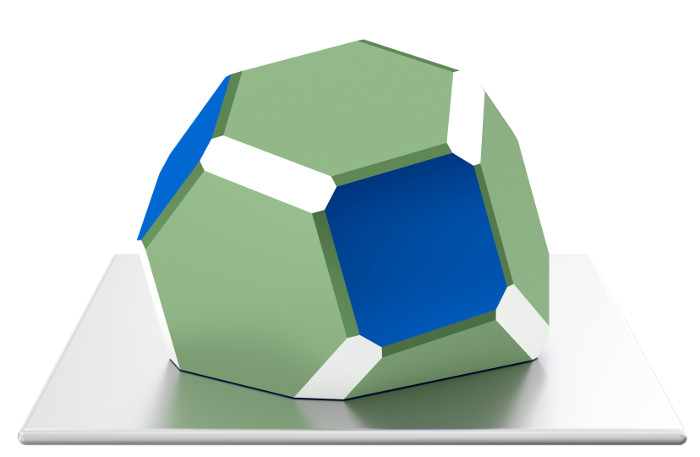A Winterbottom construction visualized with Blender.

## Source code¶

The complete source code is available in examples/graphics.py

from wulffpack import (SingleCrystal,
Decahedron,
Icosahedron)

# Define energies and create particle
surface_energies = {(1, 1, 1): 1.0,
(1, 0, 0): 1.1,
(1, 1, 0): 1.12}
particle = Decahedron(surface_energies=surface_energies,
twin_energy=0.02)

# Define colors (optional)
colors = {(1, 1, 1): '#7da275',
(1, 0, 0): '#0053b5',
(1, 1, 0): '#f6faf5'}
# Show with GUI:
particle.view()
# Save as file:
particle.view(colors=colors, linewidth=0.3, alpha=0.9,
save_as='single_particle.png')

# Use continuous color scheme
surface_energies_vicinal = {(1, 1, 1): 1.0,
(1, 1, 0): 1.1,
(1, 0, 0): 1.1,
(3, 3, 2): 1.05,
(2, 1, 0): 1.15}
particle_vicinal = SingleCrystal(surface_energies_vicinal)
continuous_colors = particle_vicinal.get_continuous_color_scheme()
particle_vicinal.view(colors=continuous_colors)

# Make figure with subplots
import matplotlib.pyplot as plt
from mpl_toolkits.mplot3d import Axes3D

fig = plt.figure(figsize=(3 * 4.0, 4.0))
particle = SingleCrystal(surface_energies)
particle.make_plot(ax, colors=colors)

particle = Decahedron(surface_energies,
twin_energy=0.05)
particle.make_plot(ax, colors=colors)

particle = Icosahedron(surface_energies,
twin_energy=0.05)
particle.make_plot(ax, colors=colors)

right=1, wspace=0, hspace=0)
plt.savefig('particles.png')
plt.show()

# Use ase.visualize.view
from ase.visualize import view
view(particle.atoms)

# Write atoms to file for further visualization
from ase.io import write
write('atoms.xyz', particle.atoms)

# Write wavefront obj file
particle.write('particle.obj')